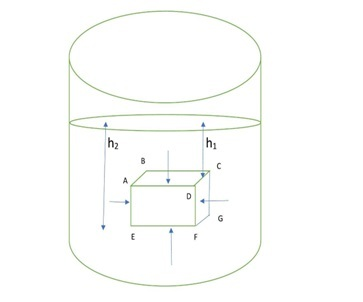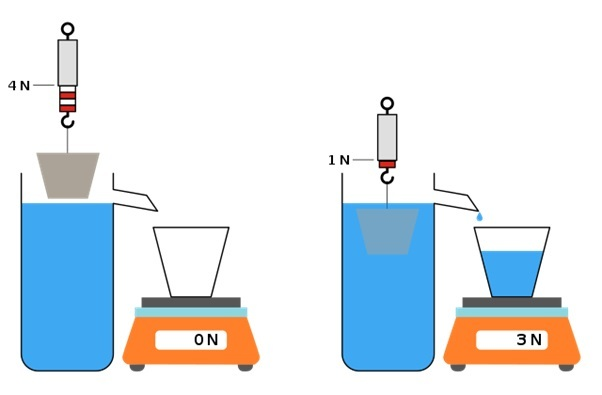# Archimedes Principle Explanation

## Introduction

Archimedes’ Principle is an important principle of fluid mechanics. It is discovered by a Greek mathematician and scientist Archimedes. This principle is utilized to estimate the volume of an object that has an irregular shape, density, and distinct gravity of an object.

## What is Archimedes' Principle?

A body placed on a fluid (liquid or gas) will experience an upward buoyant force. This force is equal to the amount of fluid displaced by the body. In the case of a completely immersed body or partially immersed body, the volume of the fluid displaced by the body will be equal to the volume of that body.

## The statement of Archimedes' Principle

If a body is totally or partly plunged in a liquid at rest, few parts of its weight appear to lose. This apparent loss of weight in the body is equal to the weight of the fluid displaced by that body.

## Archimedes' Principle Formula

Archimedes’ law of fluid states that the buoyancy force on a body due to the fluid is equal to the weight of the fluid displaced by the body. The mathematical expression of Archimedes’ law can be written as,

$$\mathrm{F=\rho Vg}$$

Here,

F= buoyant force act on immersed bogy

ρ=density of the fluid

V= volume of the displaced fluid

## Archimedes' Principle Derivation

Let us consider, a cube is immersed in a liquid of density ρ. The depth of the ABCD and EFGH surfaces of the cube from the upper surface of the liquid be $\mathrm{h_2}$ and $\mathrm{h_1,}$ respectively. The length of each side of the cube is l. The liquid exerts a normal thrust on each surface of the cube. The lateral thrust acting horizontally on the mutually opposite vertical faces ABHE and CDFG of the cube is equal and opposite.Similarly, the lateral thrust acting on the faces AEFD and BCGH balances each other. So, in the horizontal direction, the resultant lateral force is zero. But, an unequal thrust acts on the surfaces ABCD and EFGH due to the difference in depths of these surfaces from the upper side of the liquid.

The downward pressure of the liquid at any point on $\mathrm{ABCD=\rho gh_1}$

The downward thrust on $\mathrm{ABCD=l^2\:\rho gh_1}$

The upward pressure of the liquid at any point on $\mathrm{EFGH=\rho\:gh_2}$

Then, The upward thrust on $\mathrm{EFGH=l^2 \rho gh_2}$

Since $\mathrm{h_2 > h_1}$, the upward thrust acting on the cube is greater than the downward thrust.

Then,

net upward thrust $\mathrm{l^2\:\rho gh_2-l^2 ρgh_1=l^2 \rho g(h_2-h_1)=l^3 \rho g }$

Since, $\mathrm{h_2-h_1=l }$

But, $\mathrm{l^3=V (volume\:of\:the\:cube)}$

Hence,

$$\mathrm{F = l^3\:\rho\:g=V\:\rho g}$$

So, the buoyancy force on the cube = weight of the liquid displaced by the cube.

## Laws of Floating

There are three laws of flotation. So, let W is the weight of the body and w is the buoyant force.

• If W>w, i.e., the weight of the body is greater than buoyant force then the body will go down to the bottom of the liquid.

• If W<w, i.e., the weight of is less than buoyant force then the body will float partially submerged in the liquid

• If W=w, i.e., then the body will stay afloat in liquid if its whole volume is just immersed in liquid.

## Archimedes' Principle Examples

Example 1: A piece of wood of volume 20.5 cm3 is tied to a piece of lead of volume 1 cm3. State whether the combination will float or sink in water. [Specific gravity of wood and lead are respectively 0.5 and 11.4].

Ans: $\mathrm{Weight\:of\:lead=(1×11.4)gm×g=11.4gm×g}$

$\mathrm{Weight\:of\:the\:piece\:of\:wood\: =(20.5×0.5)gm×g=10.25gm×g}$

$$\mathrm{Total\:weight=21.65\:gm×g}$$

$\mathrm{Total\:Volume=(20.5+1)cm^3=21.5Cm^3}$

So, the weight of displaced water by the combination=21.5gm×g

Hence, the weight of the combination >weight of displaced water

Therefore, the combination will sink into the water.

## Archimedes' Principle Experiment

According to Archimedes’ principle,

$$\mathrm{Apparent\:weight=weight\:of\:the \:body-buoyant\:force }$$

Let’s have a look at an experiment,

• Let us take a container filled with water. The container is connected with a bowl such that if the water overflows it will displace the bowl through a pipe.

• Now take any solid object, and measure its weight by employing a spring balance in the air. Note down the value, it is 6kg.

• Next, attach the object to the spring balance and immerse it in water. The spring balance must be out of water.

• Now, as the weight is lowered some water will be enter into the bowl. The weight of the displaced water is 2 kg.

• The spring balance will display less value than previously. Note down the value which is 4 kg.

• We found that when we subtract the new weight of the body from the first measured value, it will come out equal to the weight of the displaced water.MikeRun, Archimedes-principle, CC BY-SA 4.0

## Application of Archimedes' Principle

### The upward Motion of the Balloon

The upward motion of a balloon depends on the thrust of the air. The net weight of the balloon is much less compared to the air displaced by it. Hence, the buoyant force acting on the balloon is greater than its weight. So, the balloon undergoes a resultant upward force and moves up.

### Submarine

A submarine can be submerged in water and float in water too. A submarine is comprised of counterbalance tanks and these tanks can be loaded up with air or water. At the point when the tanks are loaded up with air, the submarine floats on water in light of the fact that the heaviness of the submarine becomes lower than the heaviness of the water dislodged by it. At the point when water is permitted to enter the tanks, the heaviness of the submarine becomes more noteworthy than the heaviness of the water uprooted by it and consequently, the submarine lowers in water.

### Life Belts

A life belt is an air-filled bag. The weight of the life belt along with the weight of the person is less than the weight of the water displaced. Hence, by wearing a life belt a person can float.

## Conclusion

A body partially or fully plunged in a liquid will experience a loss of weight. This loss of weight is equivalent to the weight of the liquid shift by the body. This is known as Archimedes' Principle. This rule does not apply to a body that is in a weightless condition such as in artificial satellites or free-fall. Archimedes' Principle acts in the case of submarines, life belts, balloons, swimming, ships, etc. With the help of this Principle volume of a solid of any shape, the density of a substance, etc can be determined.

## FAQs

Q1. What is Buoyancy?

Ans. The ability of a fluid to exert an upward force at rest condition on a body immersed in that fluid is called buoyancy.

Q2. Explain whether Archimedes' Principle is applicable in the case of a free-falling body under gravity.

Ans. We know that, free-falling body is weightless. So, this Principle isn’t applicable in this case. Because a free-falling body is weightless.

Q3. An egg sinks in freshwater, but it floats in salt water. Why?

Ans. The density of an egg is higher than fresh water. So that, it sinks into the water. But, when a suitable amount of salt is added to water, the density of salt water becomes higher than an egg, and the egg floats on saline water.

Q4. Will Archimedes' Principle be applicable within an artificial satellite revolving around the sun?

Ans. All bodies become weightless inside an artificial satellite revolving around the sun. Hence, the weight of the object and the fluid displaced by the object both will be zero and the object will not experience any buoyant force. Therefore, Archimedes' Principle will not be applicable within an artificial satellite revolving around the sun.

Q5. A solid sphere and hollow sphere having the same mass and external radius are immersed in the same liquid. Which one will feel heavier?

Ans. The radius and volume of both solid and hollow spheres are equal. Hence, the liquid displaced by them will be the same. Therefore, the loss of weight in both cases will be equal and the apparent weight of both spheres will be the same.

Updated on: 18-Apr-2023

253 Views